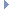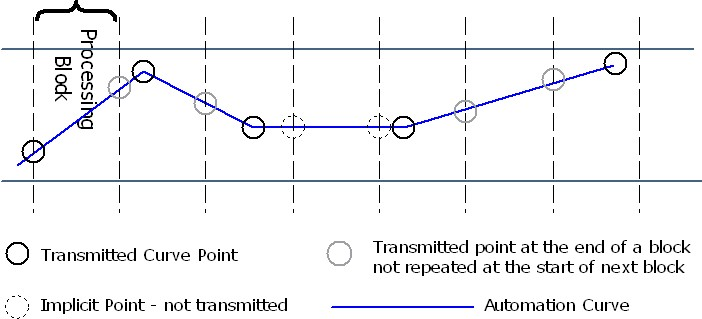VST 3 Interfaces  VST 3.7 SDK for developing VST plug-in
IParamValueQueue Class Referenceabstract

Queue of changes for a specific parameter: Vst::IParamValueQueue. More...

`#include <ivstparameterchanges.h>`Inheritance diagram for IParamValueQueue:

## Public Member Functions

virtual ParamID getParameterId ()=0
Returns its associated ID.

virtual int32 getPointCount ()=0
Returns count of points in the queue.

virtual tresult getPoint (int32 index, int32 &sampleOffset, ParamValue &value)=0
Gets the value and offset at a given index.

virtual tresult addPoint (int32 sampleOffset, ParamValue value, int32 &index)=0
Adds a new value at the end of the queue, its index is returned.Public Member Functions inherited from FUnknown
virtual tresult queryInterface (const TUID _iid, void **obj)=0

virtual uint32 release ()=0

## Static Public Attributes

static const FUID iidStatic Public Attributes inherited from FUnknown
static const FUID iid

## Detailed Description

Queue of changes for a specific parameter: Vst::IParamValueQueue.

• [host imp]
• [released: 3.0.0]
• [mandatory]

The change queue can be interpreted as segment of an automation curve. For each processing block, a segment with the size of the block is transmitted to the processor. The curve is expressed as sampling points of a linear approximation of the original automation curve. If the original already is a linear curve, it can be transmitted precisely. A non-linear curve has to be converted to a linear approximation by the host. Every point of the value queue defines a linear section of the curve as a straight line from the previous point of a block to the new one. So the plug-in can calculate the value of the curve for any sample position in the block.

Implicit Points:
In each processing block, the section of the curve for each parameter is transmitted. In order to reduce the amount of points, the point at block position 0 can be omitted.

• If the curve has a slope of 0 over a period of multiple blocks, only one point is transmitted for the block where the constant curve section starts. The queue for the following blocks will be empty as long as the curve slope is 0.
• If the curve has a constant slope other than 0 over the period of several blocks, only the value for the last sample of the block is transmitted. In this case, the last valid point is at block position -1. The processor can calculate the value for each sample in the block by using a linear interpolation:
//------------------------------------------------------------------------
double x1 = -1; // position of last point related to current buffer
double y1 = currentParameterValue; // last transmitted value
int32 pointTime = 0;
ParamValue pointValue = 0;
IParamValueQueue::getPoint (0, pointTime, pointValue);
double x2 = pointTime;
double y2 = pointValue;
double slope = (y2 - y1) / (x2 - x1);
double offset = y1 - (slope * x1);
double curveValue = (slope * bufferTime) + offset; // bufferTime is any position in buffer

Jumps:
A jump in the automation curve has to be transmitted as two points: one with the old value and one with the new value at the next sample position.## Member Function Documentation

 virtual ParamID getParameterId ( )
pure virtual

Returns its associated ID.

Implemented in ParameterValueQueue, and ParamChanges.

 virtual int32 getPointCount ( )
pure virtual

Returns count of points in the queue.

Implemented in ParameterValueQueue, and ParamChanges.

 virtual tresult getPoint ( int32 index, int32 & sampleOffset, ParamValue & value )
pure virtual

Gets the value and offset at a given index.

Implemented in ParameterValueQueue, and ParamChanges.

 virtual tresult addPoint ( int32 sampleOffset, ParamValue value, int32 & index )
pure virtual

Adds a new value at the end of the queue, its index is returned.

Implemented in ParameterValueQueue, and ParamChanges.

## Member Data Documentation

 const FUID iid
static
Empty